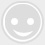# 【电子大讲堂】如何编写高效率稳定的单片机代码

-回复 -浏览21ic

1

1.使用尽量小的数据类型

• 能用unsiged就不用signed;

• 能用char就不用int;

• 能不用floating就不用。

• 能用位操作不用算数。

2.使用自加、自减指令

3.减少运算的强度

(1) 求余运算

N= N %8 可以改为N = N &7

(2) 平方运算

N=Pow(3,2) 可以改为N=3*3

(3) 用位移代替乘法除法

N=M*8 可以改为N=M<<3

N=M/8 可以改为N=M>>3

(4) 自加自减的区别

void DelayNms(UINT16 t)

{

UINT16 i,j;

for(i=0;i

define MAX(A，B) {(A)>(B)?(A):(B)}

1.适当地使用算法

UINT16 Sum(void)

{

UINT8 i,s;

for(i=1;i<=100;i++)

{

s+=i;

}

return s;

}

UINT16 Sum(void)

{

UINT16 s;

s=(100 *(100+1))>>1;

return s;

}

2.用指针代替数组

UINT8 szArrayA;

UINT8 szArrayB;

UINT8 i;

UINT8 *p=szArray;

for(i=0;i<64;i++)szArrayB[i]=szArrayA[i];

for(i=0;i<64;i++)szArrayB[i]=*p++;

3.强制转换

C 语言精髓第一精髓就是指针的使用，第二精髓就是强制转换的使用，恰当地利用指针和强制转换不但可以提供程序效率，而且使程序更加之简洁，由于强制转换在C 语言编程中占有重要的地位，下面将已五个比较典型的例子作为讲解。

UINT8 a=0;

INT8 b=-3;

a=(UINT8)b;

UINT8 a={0x12,0x34};

UINT16 b=0;

b=(a<<8)|a;

UINT8 a={0x12,0x34};

UINT16 b=0;

b= (UINT16 )a; //强制转换

typedef struct _ST

{

UINT8 a;

UINT8 b;

UINT8 c;

UINT8 d;

UINT8 e;

}ST;

ST s;

UINT8 a={0};

s.a=1;

s.b=2;

s.c=3;

s.d=4;

s.e=5;

a=s.a;

a=s.b;

a=s.c;

a=s.d;

a=s.e;

typedef struct _ST

{

UINT8 a;

UINT8 b;

UINT8 c;

UINT8 d;

UINT8 e;

}ST;

ST s;

UINT8 a={0};

UINT8 p=(UINT8 )&s;//强制转换

UINT8 i=0;

s.a=1;

s.b=2;

s.c=3;

s.d=4;

s.e=5;

for(i=0;i

define Perror(FUN) printf(“Err:%s %s %d: %s\n”, FILE, func,LINE,FUN) 类linux的perror函数实现，这里加了出错的文件位置，所在函数，引发出错调用的函数FUN。

define STR(s) #s

define CONS(a, b) int(a##e##b)

printf(STR(vck));//输出vck

printf(“%d\n”, CONS(2,3));//2e3 输出2000